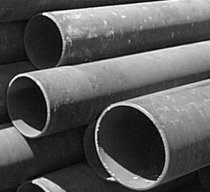Engineering ToolBox - Resources, Tools and Basic Information for Engineering and Design of Technical Applications!

# Pipe Formulas

## Pipe and Tube Equations - moment of inertia, section modulus, traverse metal area, external pipe surface and traverse internal area - imperial units

### Online Pipe Formula Calculator

outside diameter (in)

inside diameter (in) - (default values: STD 4 inches - Carbon, Alloy and Stainless Steel Pipes - ASME/ANSI B36.10/19

The calculator is based on the piping formulas and equations below.### Moment of Inertia

Moment of inertia can be expressed as

I  = π (do4 - di4) / 64
≈ 0.0491 (do4 - di4)                            (1)

where

I = moment of inertia (in4)

do = outside diameter (in)

di = inside diameter (in)

### Section Modulus

Section modulus can be expressed as

S  = 0.0982 (do4 - di4) / do                            (2)

where

S = section modulus (in3)

Section modulus is a geometric property for a given cross-section used in the design of beams or flexural members.

### Transverse Metal Area

Transverse metal area can be expressed as

Am  = π (do2 - di2) / 4                              (3)

where

Am = transverse metal area (in2)

### External Pipe Surface

External pipe or tube surface per ft of length can be expressed as

Ao  = π do / 12                              (4)

where

Ao = external  pipe surface area (ft2 per ft pipe)

### Internal Pipe Surface

Internal pipe or tube surface per ft of length can be expressed as

Ai  = π di / 12                            (5)

where

Ai = internal  pipe surface area (ft2 per ft pipe)

### Transverse Internal Area

Transverse internal area can be expressed as

Aa  = 0.7854 di2                       (6)

where

Aa = transverse internal area (in2)

### Circumference External

External circumference can be expressed as

Ce  = π do                      (7)

where

Ce =  external circumference (in)

### Circumference Internal

Internal circumference can be expressed as

Ci  = π di                       (8)

where

Ci =  internal circumference (in)

### Estimating Pipe Circumference and Section Area

Nominal Pipe Size
(in)
Circumference
(in)
Section Area
(sq.in.)
1/4 0.785 0.049
3/8 1.178 0.110
1/2 1.571 0.196
3/4 2.356 0.442
1 3.142 0.785
1 1/4 3.927 1.227
1 1/2 4.712 1.767
2 6.283 3.142
2 1/2 7.854 4.909
3 9.425 7.069
3 1/2 11.00 9.621
4 12.57 12.57
5 15.71 19.64
6 18.85 28.27
8 25.13 50.27
10 31.42 78.54
12 37.70 113.1
15 47.12 176.7

## Related Topics

• Dimensions - Sizes and dimensions of pipes and tubes, and their fittings - inside and outside diameter, weight and more.

## Engineering ToolBox - SketchUp Extension - Online 3D modeling!

Add standard and customized parametric components - like flange beams, lumbers, piping, stairs and more - to your Sketchup model with the Engineering ToolBox - SketchUp Extension - enabled for use with the amazing, fun and free SketchUp Make and SketchUp Pro .Add the Engineering ToolBox extension to your SketchUp from the SketchUp Pro Sketchup Extension Warehouse!

Translate

## Privacy

We don't collect information from our users. Only emails and answers are saved in our archive. Cookies are only used in the browser to improve user experience.

Some of our calculators and applications let you save application data to your local computer. These applications will - due to browser restrictions - send data between your browser and our server. We don't save this data.

## Citation

• Engineering ToolBox, (2008). Pipe Formulas. [online] Available at: https://www.engineeringtoolbox.com/pipe-formulas-d_1335.html [Accessed Day Mo. Year].

Modify access date.

. .

#### Scientific Online Calculator6 24